# 序列到序列 (Seq2Seq) 和注意力机制 (Attention Machanism)

## Encoder-Decoder & Seq2Seq

Encoder-Decoder 是一种包含两个神经网络的模型，两个网络分别扮演编码器和解码器的角色。Cho 等人 1 提出了一个基于 RNN 的 Encoder-Decoder 神经网络用于机器翻译。网络结构如下图所示：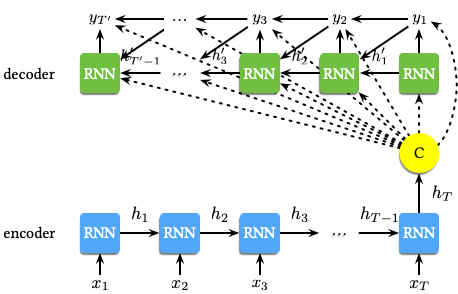$$\mathbf{h}_{\langle t \rangle} = f \left(\mathbf{h}_{\langle t-1 \rangle}, x_t\right)$$

$$\mathbf{h}'_{\langle t \rangle} = f \left(\mathbf{h}'_{\langle t-1 \rangle}, y_{t-1}, \mathbf{c}\right)$$

$$P \left(y_t | y_{t-1}, y_{t-2}, \dotsc, y_1, \mathbf{c}\right) = g \left(\mathbf{h}_{\langle t \rangle}, y_{t-1}, \mathbf{c}\right)$$

RNN Encoder-Decoder 的两部分通过最大化如下的对数似然函数的联合训练进行优化：

$$\max_{\theta} \dfrac{1}{N} \sum_{n=1}^{N}{\log p_{\theta} \left(\mathbf{y}_n | \mathbf{x}_n\right)}$$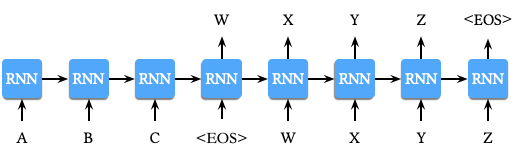1. 对数据进行预处理，在每个句子的结尾添加特殊字符 <EOS>，如上图所示。首先计算 A, B, C, <EOS> 的表示，再利用该表示计算 W, X, Y, Z, <EOS> 的条件概率。
2. 利用两个不同的 LSTM，一个用于输入序列，另一个用于输出序列。
3. 选用一个较深的 LSTM 模型 (4 层) 提升模型效果。
4. 对输入序列进行倒置处理，例如对于输入序列 $a, b, c$ 和对应的输出序列 $\alpha, \beta, \gamma$，LSTM 需要学习的映射关系为 $c, b, a \to \alpha, \beta, \gamma$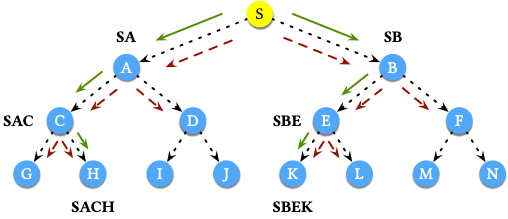1. 第一步搜索的可能结果为 SA 和 SB，保留 Top 2，结果为 SA 和 SB。
2. 第二步搜索的可能结果为 SAC，SAD，SBE 和 SBF，保留 Top 2，结果为 SAC 和 SBE。
3. 第三步搜索的可能结果为 SACG，SACH，SBEK 和 SBEL，保留 Top 2，结果为 SACH 和 SBEK。至此，整个搜索结束。

Bahdanau 等人 3 提出了一种基于双向 RNN (Bidirectional RNN, BiRNN) 结合注意力机制 (Attention Mechanism) 的网络结构用于机器翻译。网络结构如下：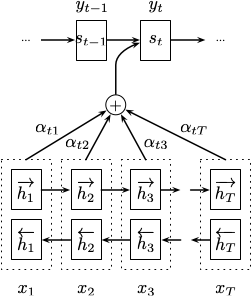$$p \left(y_i | y_1, \dotsc, y_{i-1}, \mathbf{x}\right) = g \left(y_{i-1}, s_i, c_i\right)$$

$$s_i = f \left(s_{i-1}, y_{i-1}, c_i\right)$$

## 注意力机制 (Attention Mechanism)

Bahdanau 等人在文中 3 提出传统的 Encoder-Decoder 模型将输入序列压缩成一个固定长度的向量 $c$，但当输入的序例很长时，尤其是当比训练集中的语料还长时，模型的的效果会显著下降。针对这个问题，如上文所述，上下文向量 $c_i$ 依赖于 $\left(h_1, \dotsc, h_T\right)$。其中，每个 $h_i$ 都包含了整个序列的信息，同时又会更多地关注第 $i$ 个词附近的信息。对于 $c_i$，计算方式如下：

$$c_i = \sum_{j=1}^{T}{\alpha_{ij} h_j}$$

$$\alpha_{ij} = \dfrac{\exp \left(e_{ij}\right)}{\sum_{k=1}^{T}{\exp \left(e_{ik}\right)}}$$

$$a \left(s_{i-1}, h_j\right) = v_a^{\top} \tanh \left(W_a s_{i-1} + U_a h_j\right)$$

### Hard & Soft Attention

Xu 等人 4 在图像标题生成 (Image Caption Generation) 任务中引入了注意力机制。在文中作者提出了 Hard Attenttion 和 Soft Attention 两种不同的注意力机制。

$$\begin{equation} \begin{split} & p \left(s_{t, i} = 1 | s_{j < t}, \mathbf{a}\right) = \alpha_{t, i} \\ & \hat{\mathbf{z}}_t = \sum_{i}{s_{t, i} \mathbf{a}_i} \end{split} \end{equation}$$

$$\mathbb{E}_{p \left(s_t | a\right)} \left[\hat{\mathbf{z}}_t\right] = \sum_{i=1}^{L}{\alpha_{t, i} \mathbf{a}_i}$$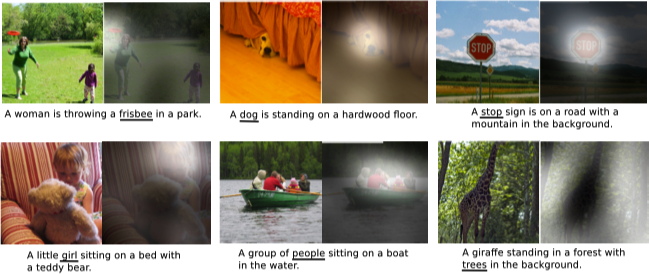### Global & Local Attention

Luong 等人 5 提出了 Global Attention 和 Local Attention 两种不同的注意力机制用于机器翻译。Global Attention 的思想是在计算上下文向量 $c_t$ 时将编码器的所有隐状态均考虑在内。对于对齐向量 $\boldsymbol{a}_t$，通过比较当前目标的隐状态 $\boldsymbol{h}_t$ 与每一个输入的隐状态 $\bar{\boldsymbol{h}}_s$ 得到，即：

\begin{equation} \begin{split} \boldsymbol{a}_t &= \text{align} \left(\boldsymbol{h}_t, \bar{\boldsymbol{h}}_s\right) \\ &= \dfrac{\exp \left(\text{score} \left(\boldsymbol{h}_t, \bar{\boldsymbol{h}}_s\right)\right)}{\sum_{s'}{\exp \left(\text{score} \left(\boldsymbol{h}_t, \bar{\boldsymbol{h}}_{s'}\right)\right)}} \end{split} \end{equation}

$$\text{score} \left(\boldsymbol{h}_t, \bar{\boldsymbol{h}}_s\right) = \begin{cases} \boldsymbol{h}_t^{\top} \bar{\boldsymbol{h}}_s & dot \\ \boldsymbol{h}_t^{\top} \boldsymbol{W}_a \bar{\boldsymbol{h}}_s & general \\ \boldsymbol{W}_a \left[\boldsymbol{h}_t; \bar{\boldsymbol{h}}_s\right] & concat \end{cases}$$

$$\boldsymbol{a}_t = \text{softmax} \left(\boldsymbol{W}_a \boldsymbol{h}_t\right)$$

Global Attention 模型的网络结构如下所示：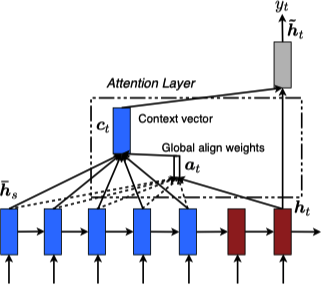Global Attention 的一个问题在于任意一个输出都需要考虑输入端的所有隐状态，这对于很长的文本 (例如：一个段落或一篇文章) 计算量太大。Local Attention 为了解决这个问题，首先在 $t$ 时刻对于每个目标词生成一个对齐位置 $p_t$，其次上下文向量 $\boldsymbol{c}_t$ 则由以 $p_t$ 为中心前后各 $D$ 大小的窗口 $\left[p_t - D, p_t + D\right]$ 内的输入的隐状态计算得到。不同于 Global Attention，Local Attention 的对齐向量 $\boldsymbol{a}_t \in \mathbb{R}^{2D + 1}$ 为固定维度。

$$p_t = S \cdot \text{sigmoid} \left(\boldsymbol{v}_p^{\top} \tanh \left(\boldsymbol{W}_p \boldsymbol{h}_t\right)\right)$$

\boldsymbol{a}_t \left(s\right) = \text{align} \left(\boldsymbol{h}_t, \bar{\boldsymbol{h}}_s\right) \exp \left(- \dfrac{\left(s - p_t\right)^2}{2 \sigma^2}\right)

Local Attention 模型的网络结构如下所示：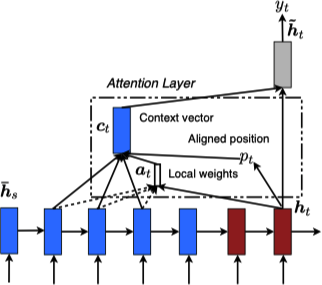### Self Attention

Vaswani 等人 6 提出了一种新的网络结构，称之为 Transformer，其中采用了自注意力 (Self-attention) 机制。自注意力是一种将同一个序列的不同位置进行自我关联从而计算一个句子表示的机制。Transformer 利用堆叠的 Self Attention 和全链接网络构建编码器 (下图左) 和解码器 (下图右)，整个网络架构如下图所示：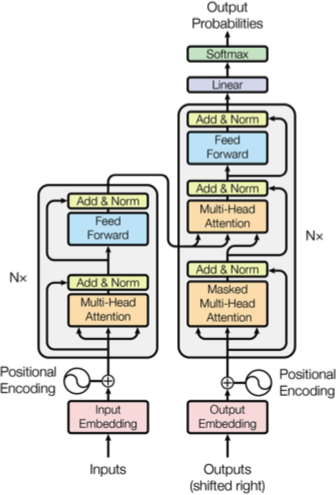#### Scaled Dot-Product & Multi-Head Attention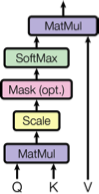$$\text{Attention} \left(Q, K, V\right) = \text{softmax} \left(\dfrac{Q K^{\top}}{\sqrt{d_k}}\right) V$$

Multi-Head Attention 的做法并不直接对原始的 keys，values 和 queries 应用注意力函数，而是学习一个三者各自的映射再应用 Atteneion，同时将这个过程重复 $h$ 次。Multi-Head Attention 的网路结构如下图所示：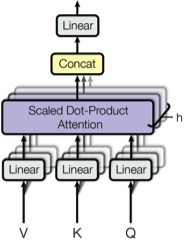$$\begin{equation} \begin{split} \text{MultiHead} \left(Q, K, V\right) &= \text{Concat} \left(\text{head}_1, \dotsc, \text{head}_h\right) W^O \\ \textbf{where } \text{head}_i &= \text{Attention} \left(QW_i^Q, KW_i^K, VW_i^V\right) \end{split} \end{equation}$$

1. Encoder-Decoder Attention Layers，其中 queries 来自于之前的 Decoder 层，keys 和 values 来自于 Encoder 的输出，该部分同其他 Seq2Seq 模型的 Attention 机制类似。
2. Encoder Self-Attention Layers，其中 queries，keys 和 values 均来自之前的 Encoder 层的输出，同时 Encoder 层中的每个位置都能够从之前层的所有位置获取到信息。
3. Decoder Self-Attention Layers，其中 queries，keys 和 values 均来自之前的 Decoder 层的输出，但 Decoder 层中的每个位置仅可以从之前网络层的包含当前位置之前的位置获取信息。

#### Position-wise Feed-Forward Networks

$$\text{FFN} \left(x\right) = \max \left(0, x W_1 + b_1\right) W_2 + b_2$$

#### Positional Encoding

Transformer 模型由于未使用任何循环和卷积组件，因此为了利用序列的位置信息则在模型的 Embedding 输入中添加了 Position Encoding。Position Encoding 的维度同 Embedding 的维度相同，从而可以与 Embedding 进行加和，文中使用了如下两种形式：

$$\begin{equation} \begin{split} PE_{\left(pos, 2i\right)} &= \sin \left(pos / 10000^{2i / d_{\text{model}}}\right) \\ PE_{\left(pos, 2i+1\right)} &= \cos \left(pos / 10000^{2i / d_{\text{model}}}\right) \end{split} \end{equation}$$

$$\begin{equation} \begin{split} \sin \left(\alpha + \beta\right) &= \sin \alpha \cos \beta + \cos \alpha \sin \beta \\ \cos \left(\alpha + \beta\right) &= \cos \alpha \sin \beta - \sin \alpha \sin \beta \end{split} \end{equation}$$

#### Why Self-Attention

Self-Attention $O \left(n^2 \cdot d\right)$ $O \left(1\right)$ $O \left(1\right)$
Recurrent $O \left(n \cdot d^2\right)$ $O \left(n\right)$ $O \left(n\right)$
Convolutional $O \left(k \cdot n \cdot d^2\right)$ $O \left(1\right)$ $O \left(\log_k \left(n\right)\right)$
Self-Attention (restricted) $O \left(r \cdot n \cdot d\right)$ $O \left(1\right)$ $O \left(n/r\right)$
1. 对于每层的复杂度，当序例的长度 $n$ 比表示的维度 $d$ 小时，Self-Attention 要比循环结构计算复杂度小。为了改进在长序列上 Self-Attention 的计算性能，Self-Attention 可以被限制成仅考虑与输出位置对应的输入序列位置附近 $r$ 窗口大小内的信息。
2. Recurrent 层的最小序列操作数为 $O \left(n\right)$，其他情况为 $O \left(1\right)$，这使得 Recurrent 的并行能力较差，即上表中的 Self-Attention (restricted)。
3. 学习到长距离依赖是很多序列任务的关键，影响该能力的一个重要因素就是前向和后向信号穿越整个网络的路径长度，这个路径长度越短，越容易学习到长距离依赖。

#### Attention Visualizations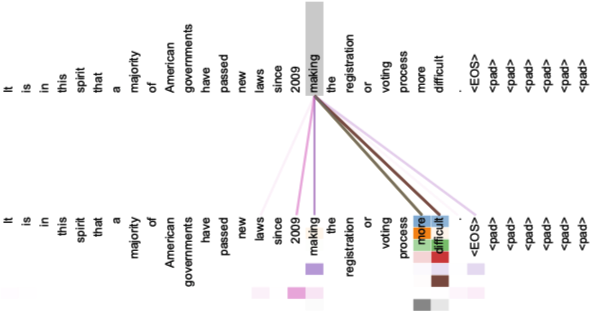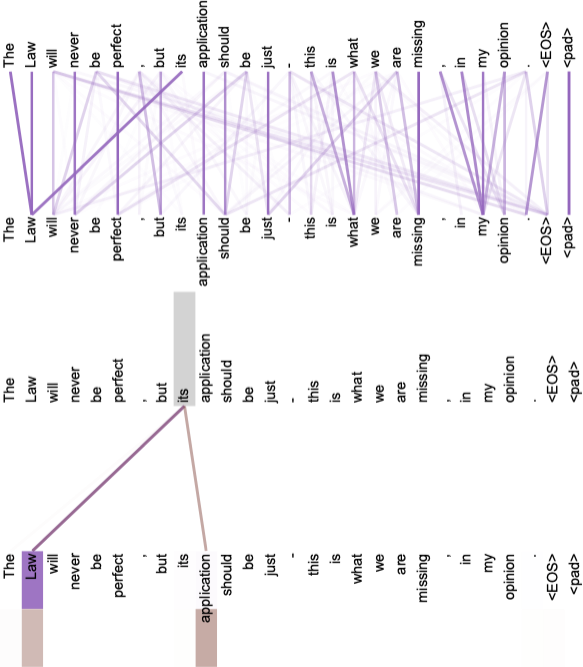### Hierarchical Attention

Yang 等人 9 提出了一种层级的注意力 (Hierarchical Attention) 网络用于文档分类。Hierarchical Attention 共包含 4 层：一个词编码器 (Word Encoder)，一个词级别的注意力层 (Word Attention)，一个句子编码器 (Sentence Encoder) 和一个句子级别的注意力层 (Sentence Attention)。网络架构如下图所示：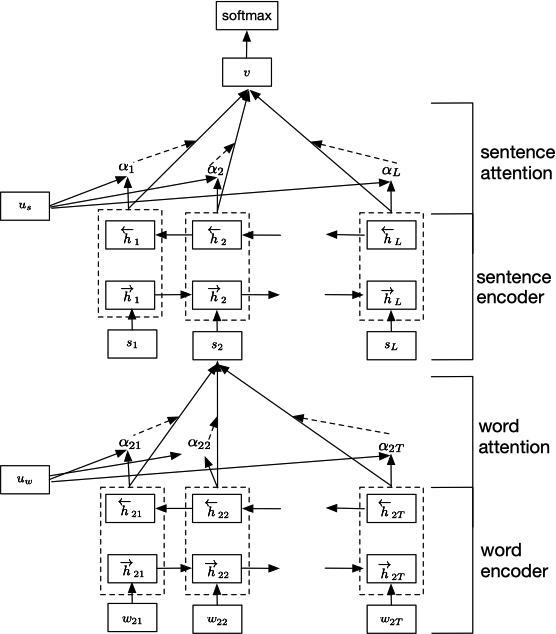#### Word Encoder

$$\begin{equation} \begin{split} x_{it} &= W_e w_{it}, t \in \left[1, T\right] \\ \overrightarrow{h}_{it} &= \overrightarrow{\text{GRU}} \left(x_{it}\right), t \in \left[1, T\right] \\ \overleftarrow{h}_{it} &= \overleftarrow{\text{GRU}} \left(x_{it}\right), t \in \left[T, 1\right] \end{split} \end{equation}$$

#### Word Attention

Word Attention 同一般的 Attention 机制类似，计算方式如下：

$$\begin{equation} \begin{split} u_{it} &= \tanh \left(W_w h_{it} + b_w\right) \\ a_{it} &= \dfrac{\exp \left(u_{it}^{\top} u_w\right)}{\sum_{t}{\exp \left(u_{it}^{\top} u_w\right)}} \\ s_i &= \sum_{t}{a_{it} h_{it}} \end{split} \end{equation}$$

#### Sentence Encoder

$$\begin{equation} \begin{split} \overrightarrow{h}_i &= \overrightarrow{\text{GRU}} \left(s_i\right), i \in \left[1, L\right] \\ \overleftarrow{h}_i &= \overleftarrow{\text{GRU}} \left(s_i\right), i \in \left[L, 1\right] \end{split} \end{equation}$$

#### Sentence Attention

$$\begin{equation} \begin{split} u_i &= \tanh \left(W_s h_i + b_s\right) \\ a_i &= \dfrac{\exp \left(u_i^{\top} u_s\right)}{\sum_{i}{\exp \left(u_i^{\top} u_s\right)}} \\ v &= \sum_{i}{a_i h_i} \end{split} \end{equation}$$

### Attention-over-Attention

Cui 等人 10 提出了 Attention-over-Attention 的模型用于阅读理解 (Reading Comprehension)。网络结构如下图所示：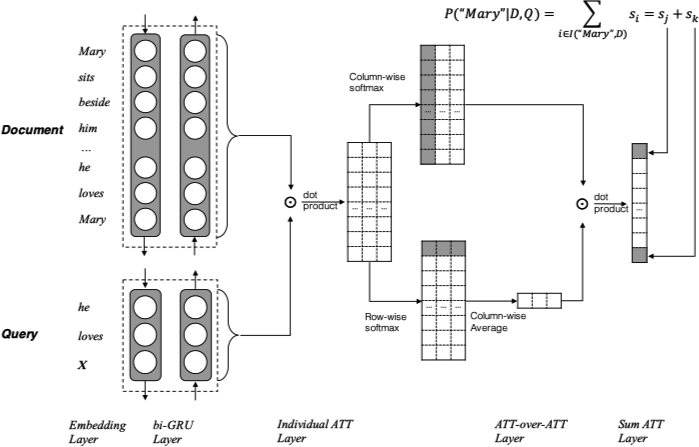1. 先获取 Document 和 Query 的 Embedding 结果，再应用一个双向的 GRU 得到对应的隐状态 $h_{doc}$$h_{query}$
2. 计算一个 Document 和 Query 的匹配程度矩阵 $M \in \mathbb{R}^{\lvert \mathcal{D} \rvert \times \lvert \mathcal{Q} \rvert}$，其中第 $i$ 行第 $j$ 列的值计算方式如下： $$M \left(i, j\right) = h_{doc} \left(i\right)^{\top} \cdot h_{query} \left(j\right)$$
3. 按照 的方向对矩阵 $M$ 应用 softmax 函数，矩阵中的每一列为考虑一个 Query 中的词的 Document 级别的 Attention，因此定义 $\alpha \left(t\right) \in \mathbb{R}^{\lvert \mathcal{D} \rvert}$$t$ 时刻的 Document 级别 Attention (query-to-document attention)。计算方式如下： $$\begin{equation} \begin{split} \alpha \left(t\right) &= \text{softmax} \left(M \left(1, t\right), \dotsc, M \left(\lvert \mathcal{D} \rvert, t\right)\right) \\ \alpha &= \left[\alpha \left(1\right), \alpha \left(2\right), \dotsc, \alpha \left(\lvert \mathcal{Q} \rvert\right)\right] \end{split} \end{equation}$$
4. 同理按照 的方向对矩阵 $M$ 应用 softmax 函数，可以得到 $\beta \left(t\right) \in \mathbb{R}^{\lvert \mathcal{Q} \rvert}$$t$ 时刻的 Query 级别的 Attention (document-to-query attention)。计算方式如下： $$\beta \left(t\right) = \text{softmax} \left(M \left(t, 1\right), \dotsc, M \left(t, \lvert \mathcal{Q} \rvert\right)\right)$$
5. 对于 document-to-query attention，我们对结果进行平均得到： $$\beta = \dfrac{1}{n} \sum_{t=1}^{\lvert \mathcal{D} \rvert}{\beta \left(t\right)}$$
6. 最终利用 $\alpha$$\beta$ 的点积 $s = \alpha^{\top} \beta \in \mathbb{R}^{\lvert \mathcal{D} \rvert}$ 得到 attended document-level attention (即 attention-over-attention)。

### Multi-step Attention

Gehring 等人 11 提出了基于 CNN 和 Multi-step Attention 的模型用于机器翻译。网络结构如下图所示：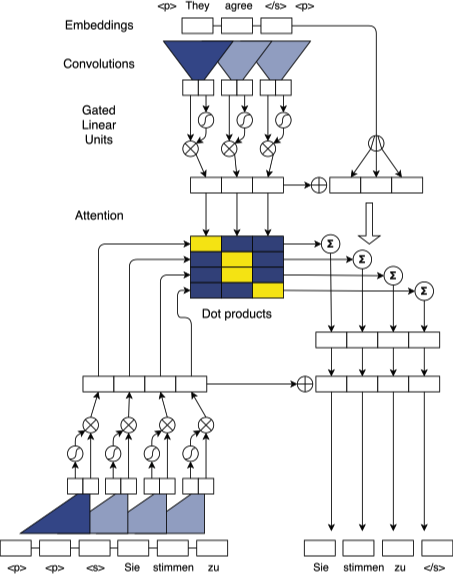#### Convolutional Block Structure

$$v \left(\left[A, B\right]\right) = A \otimes \sigma \left(B\right)$$

$$h_i^l = v \left(W^l \left[h_{i-k/2}^{l-1}, \dotsc, h_{i+k/2}^{l-1}\right] + b_w^l\right) + h_i^{l-1}$$

$$p \left(y_{i+1} | y_1, \dotsc, y_i, \mathbf{x}\right) = \text{softmax} \left(W_o h_i^L + b_o\right) \in \mathbb{R}^T$$

#### Multi-step Attention

$$d_i^l = W_d^l h_i^l + b_d^l + g_i$$

$$a_{ij}^l = \dfrac{\exp \left(d_i^l \cdot z_j^u\right)}{\sum_{t=1}^{m}{\exp \left(d_i^l \cdot z_t^u\right)}}$$

$$c_i^l = \sum_{j=1}^{m}{a_{ij}^l \left(z_j^u + e_j\right)}$$

1. Cho, K., van Merrienboer, B., Gulcehre, C., Bahdanau, D., Bougares, F., Schwenk, H., & Bengio, Y. (2014). Learning Phrase Representations using RNN Encoder–Decoder for Statistical Machine Translation. In Proceedings of the 2014 Conference on Empirical Methods in Natural Language Processing (EMNLP) (pp. 1724–1734). ↩︎

2. Sutskever, I., Vinyals, O., & Le, Q. V. (2014). Sequence to Sequence Learning with Neural Networks. In Z. Ghahramani, M. Welling, C. Cortes, N. D. Lawrence, & K. Q. Weinberger (Eds.), Advances in Neural Information Processing Systems 27 (pp. 3104–3112). ↩︎

3. Bahdanau, D., Cho, K., & Bengio, Y. (2014). Neural Machine Translation by Jointly Learning to Align and Translate. arXiv preprint arXiv:1409.0473 ↩︎ ↩︎ ↩︎

4. Xu, K., Ba, J., Kiros, R., Cho, K., Courville, A., Salakhudinov, R., … Bengio, Y. (2015). Show, Attend and Tell: Neural Image Caption Generation with Visual Attention. In International Conference on Machine Learning (pp. 2048–2057). ↩︎

5. Luong, T., Pham, H., & Manning, C. D. (2015). Effective Approaches to Attention-based Neural Machine Translation. In Proceedings of the 2015 Conference on Empirical Methods in Natural Language Processing (pp. 1412–1421). ↩︎

6. Vaswani, A., Shazeer, N., Parmar, N., Uszkoreit, J., Jones, L., Gomez, A. N., … Polosukhin, I. (2017). Attention is All you Need. In I. Guyon, U. V. Luxburg, S. Bengio, H. Wallach, R. Fergus, S. Vishwanathan, & R. Garnett (Eds.), Advances in Neural Information Processing Systems 30 (pp. 5998–6008). ↩︎

7. He, K., Zhang, X., Ren, S., & Sun, J. (2016). Deep Residual Learning for Image Recognition. In 2016 IEEE Conference on Computer Vision and Pattern Recognition (CVPR) (pp. 770–778). ↩︎

8. Ba, J. L., Kiros, J. R., & Hinton, G. E. (2016). Layer Normalization. arXiv preprint arXiv:1607.06450 ↩︎

9. Yang, Z., Yang, D., Dyer, C., He, X., Smola, A., & Hovy, E. (2016). Hierarchical Attention Networks for Document Classification. In Proceedings of the 2016 Conference of the North American Chapter of the Association for Computational Linguistics: Human Language Technologies (pp. 1480–1489). ↩︎

10. Cui, Y., Chen, Z., Wei, S., Wang, S., Liu, T., & Hu, G. (2017). Attention-over-Attention Neural Networks for Reading Comprehension. In Proceedings of the 55th Annual Meeting of the Association for Computational Linguistics (Volume 1: Long Papers) (pp. 593–602). ↩︎

11. Gehring, J., Auli, M., Grangier, D., Yarats, D., & Dauphin, Y. N. (2017). Convolutional Sequence to Sequence Learning. In International Conference on Machine Learning (pp. 1243–1252). ↩︎

12. Dauphin, Y. N., Fan, A., Auli, M., & Grangier, D. (2016). Language Modeling with Gated Convolutional Networks. arXiv preprint arXiv:1612.08083 ↩︎

13. Sukhbaatar, S., szlam, arthur, Weston, J., & Fergus, R. (2015). End-To-End Memory Networks. In C. Cortes, N. D. Lawrence, D. D. Lee, M. Sugiyama, & R. Garnett (Eds.), Advances in Neural Information Processing Systems 28 (pp. 2440–2448). ↩︎